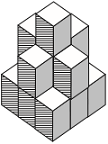# User ForumSubject :IMO    Class : Class 6

Count the number of cubes in the given figure.A8
B9
C12
D15

there is only 13....

## Ans 1: (Master Answer)

Class : Class 1

(D): Number of cubes in top layer = 1
Number of cubes in middle layer = 5
Number of cubes in bottom layer = 9
Total number of cubes = 1 + 5 + 9 = 15